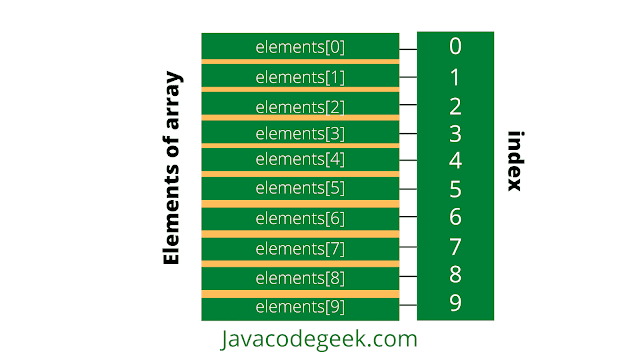-->

Single Dimensional Array In Java 

An array is a collection of similar type of elements which has contiguous memory location.

Single Dimensional Array in java is always used with only one subscript([]). A Single dimensional array behaves likes a list of variables.

Array in Java is index-based, the first element of the array is stored at the 0th index, the 2nd element is stored on 1st index, and so on.

Declaration of Single dimensional array in java

To use anything in java, we must first declare it so to use array as well, we must declare it. Like normal variables, we must provide the data type of array and name of an array.

Data type means the type of data we want to store in it. Then a name through which we will access the array elements.
datatype[] arrayName;
Or
datatype arrayName[];
Or
datatype []arrayName;
datatype -- It is either primitive data type(int, char, double, byte, etc.)or Non-primitive data (Objects).
arrayName -- is the name of an array [] is called subscript.

Example:

int[] elements;
Or
int elements[];
Or
int []elements;

Construction of Single Dimensional Array in java

To create an array new keyword is used with a data type of array. we must declare the size of the array. The size should be an integer value or a variable that contains an integer value. Size variable defines how many elements we can store in an array.
nameOfArray = new DataType[size];
Here nameOfArray is the reference variable through which we will access the array values with index passed like nameOfArray to access the first value with 0th index.

Example:

int[] elements =  new int;
In this above example new int creates a new array of type int in heap memory. The assignment operator assigns the array object to the reference variable elements

Now We can access the array by use of the elements variable. We can access each value of the array by using elements with subscript([]).

We must have to use only one subscript([]) for a single dimensional array in java.

When we create a new array the values in the array elements will automatically be initialized by their default values. For e.g. : zero (int types), false (boolean), float(0.0) or null (for object types).Array indexing starts from 0 and ends with length-1. The first element of an array is elements, the second is elements and so on.

If the length of an array is n, the last element will be arrayName[length-1].
Since the length of the elements array is 10, the last element of the array is elements.

Initialization of Single Dimensional Array in java

We can construct and initialize an array while declaring it with the syntax defined below
dataType arrayName[] = {value1, value2, …valueN}

Few important points about an array

1. The type of value which array will have should be similar to a datatype which means if we are creating int type array then it can only store int values. If we try to store different type values it will give a compile-time error.
2. Values in the array are zero-index based which means the first value will be stored at the 0th index.
3. The size of the array totally depends on the values you are providing at the time of the creation of the array.

Example:

int  number[] = {10, 20, 30, 40, 50, 60, 70, 80, 90, 100};

In this example, we have created an array of int type as you see we are initializing it during declaration only.

Example of Single dimensional array in java

import java.util.Arrays;

public class SingleDimensionalArray {
public static void main(String[] args) {
int[] elements = new int[] { 1, 2, 3, 4, 5, 6, 7, 8, 9, 10 };
System.out.println(Arrays.toString(elements));
}
}

Output:

[1, 2, 3, 4, 5, 6, 7, 8, 9, 10]
Here we have used the Arrays.toString() method to print our array.

how to Print Single dimensional array in java Using For loop

import java.util.Arrays;

public class SingleDimensionalArray {
public static void main(String[] args) {
int[] elements = new int[] { 1, 2, 3, 4, 5, 6, 7, 8, 9, 10 };
for (int i = 0; i < elements.length; i++) {
System.out.println(elements[i]);
}
}
}

Output:

1
2
3
4
5
6
7
8
9
10
Here we have used the normal for loop to print our array.

Conclusion:

In this tutorial, we have covered Single Dimensional Array in Java or One Dimensional Array in Java with memory representation in heap.

Thanks for reading this tutorial so far. If you like this tutorial then please share it with your friends and colleagues. If you have any questions, doubts, suggestions, or feedback then please drop a comment and I'll try to answer your question.

Happy Learning!!!